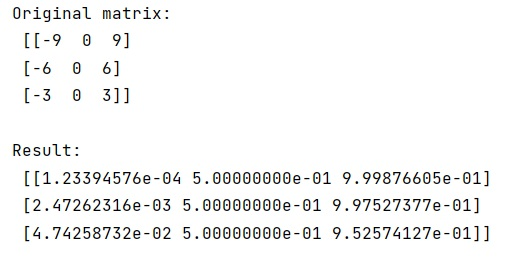# How to apply a function / map values of each element in a 2d numpy array/matrix?

Learn, how to apply a function / map values of each element in a 2d numpy array/matrix?
Submitted by Pranit Sharma, on January 24, 2023

NumPy is an abbreviated form of Numerical Python. It is used for different types of scientific operations in python. Numpy is a vast library in python which is used for almost every kind of scientific or mathematical operation. It is itself an array which is a collection of various methods and functions for processing the arrays.

## Applying a function / map values of each element in a 2d numpy array/matrix

Suppose that we are given a NumPy matrix, and, we have a self-defined function. We need to get a new array where each element is the result of the function defined by us so we need to apply this function to each value of the matrix.

Apparently, the way to apply a function to elements is to convert our function into a vectorized version that takes arrays as input and returns arrays as output. We can easily convert your function to vectorized form using numpy.vectorize().

Let us understand with the help of an example,

## Python code to apply a function / map values of each element in a 2d numpy array/matrix

```# Import numpy
import numpy as np

# Import math
import math

# Creating a numpy matrix
mat = np.matrix('-9 0 9; -6 0 6; -3 0 3')

# Display original matrix
print("Original matrix:\n",mat,"\n")

# defining a function
def fun(x):
return 1 / (1 + math.exp(-x))

# Vectorizing function
func_vec = np.vectorize(fun)

# Applying function
res = func_vec(mat)

# Display result
print("Result:\n",res,"\n")
```

Output:What's New (MCQs)

Top Interview Coding Problems/Challenges!

IncludeHelp's Blogs

Languages: » C » C++ » C++ STL » Java » Data Structure » C#.Net » Android » Kotlin » SQL
Web Technologies: » PHP » Python » JavaScript » CSS » Ajax » Node.js » Web programming/HTML
Solved programs: » C » C++ » DS » Java » C#
Aptitude que. & ans.: » C » C++ » Java » DBMS
Interview que. & ans.: » C » Embedded C » Java » SEO » HR
CS Subjects: » CS Basics » O.S. » Networks » DBMS » Embedded Systems » Cloud Computing
» Machine learning » CS Organizations » Linux » DOS
More: » Articles » Puzzles » News/Updates